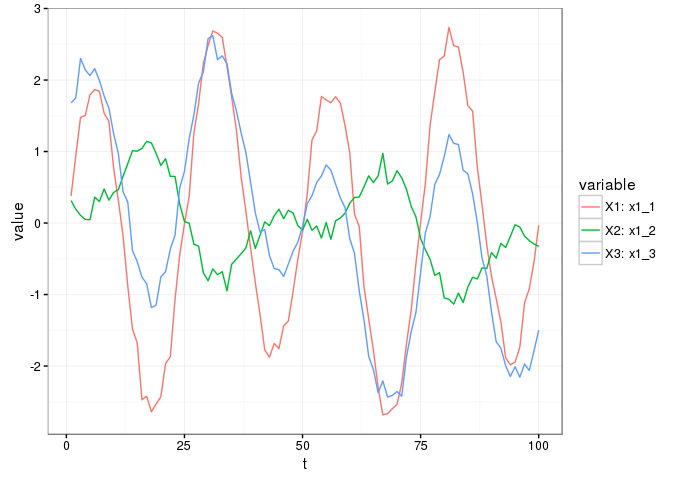COCOREG is an R package for extracting shared variation between datasets using regression models. The details of the algorithm are to be described in a paper:

Korpela J, Henelius A, Ahonen L, Klami A, Puolamäki K (2016) Using regression makes extraction of shared variation in multiple datasets easy. Data Mining And Knowledge Discovery, submitted 2015.

In short, a chain of regression models is used to “filter” the data such that the output contains only variation that is shared by all input datasets. The shared variation is presented in the same space as the input data i.e. using the same variables as the input data.

## Basic usage

The following is a minimal usage example. It creates a toy data collection (i.e. a set of datasets), runs cocoreg on it and visualizes:

library(cocoreg)
dc <- create_syn_data_toy()
ccr <- cocoreg(dc$data) shared.by.all.df <- variation_shared_by(dc, 'all') #only on synthetic datasets ggplot_dflst(dc$data, ncol = 1)
ggplot_dflst(ccr$data, ncol = 1)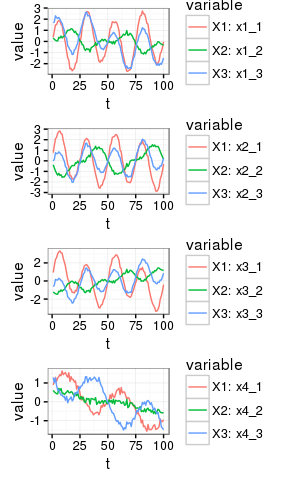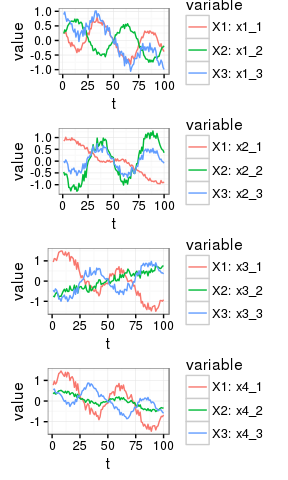To plot several data collections sidy-by-side use: ggplot_dclst(list(observed = dc$data, shared = shared.by.all.df, cocoreg = ccr$data))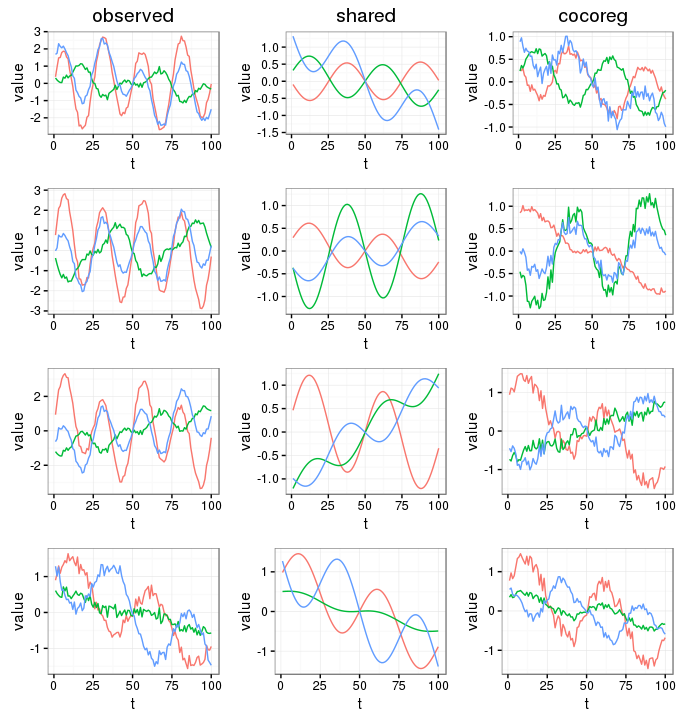To compare two or more data collections variable by variable: library(reshape) #importing from namespace does not work as expected ggcompare_dclst(list(shared = shared.by.all.df, cocoreg = ccr$data))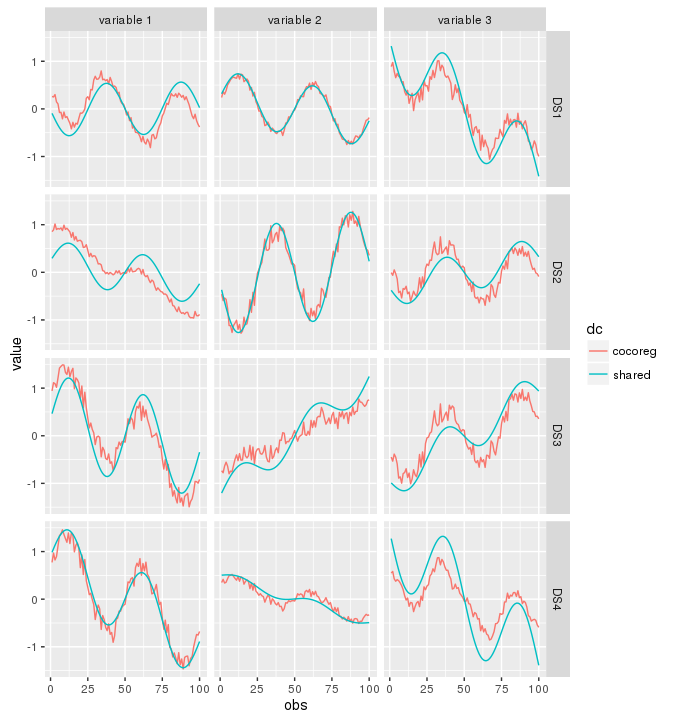Other visualizations:

ggplot_dclst(list(observed = dc$data, shared = shared.by.all.df, cocoreg = ccr$data),
legendMode = 'all')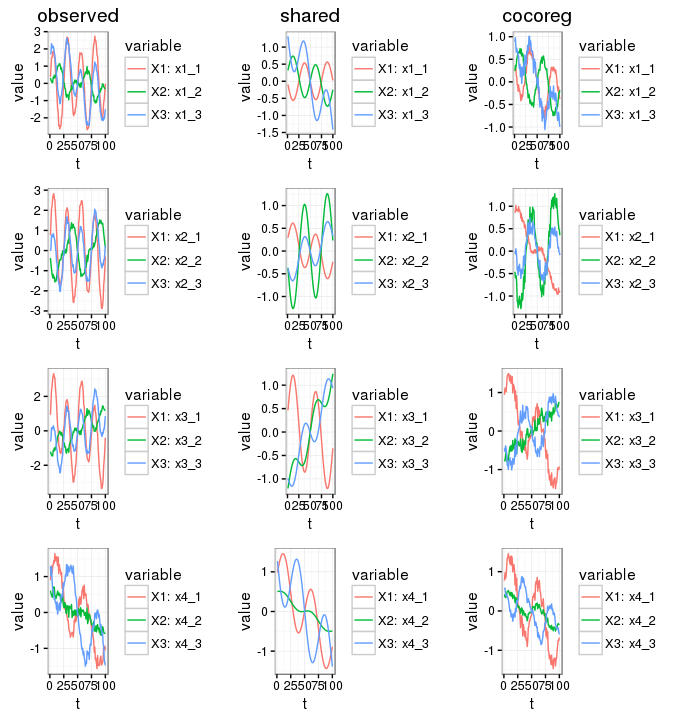ggplot_dflst(dc$data, ncol=1)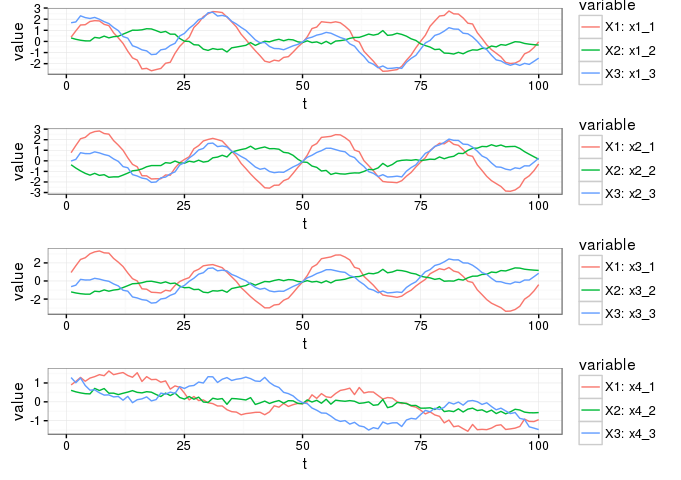ggplot_df(dc$data[])